Table ratio measurement

The brilliant is observed under the microscope at a chosen magnification at right-angles to the plane of the table. The stone should not be tilted. As a check, the girdle, which lies in a plane parallel to the table, should be sharply focused right round. One of the eight table edges serves as the first reference line (A) (Fig 209).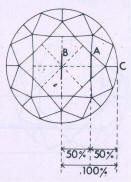Parallel to it, through the centre of the stone runs one of the edges of the eight pavilion girdle facets; it forms the second reference line (B). Parallel to both these lines a third line (C) is, imagined as a tangent touching the girdle at point X. First of all the line of the table-edge A is taken as the only visible reference line and then the distances AB and AC are compared with one another, so then. at a ratio of 50: 50, the table size = 50 %.
As this estimate starts from the table edge instead-of from a table corner,
which lies further away from the centre than the edge, 4 % must be added tc the result.

Thus the table size is actually 50 % + 4 % = 54 % of the girdle diameter.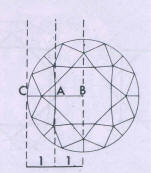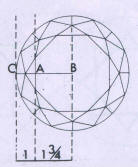Fig 210 Measuring table in relation to visual estimation of the size of the table

From this observation further relatives values result:

AS : AC = table size 1 1/4: 1 = 60 %
1 1/2: 1 = 65 %
1 3/4: 1 = 69 %
2 :1=72%

 54% 1:1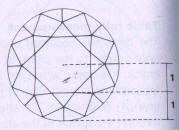60% 1¼:1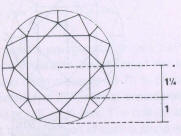65% 1½:1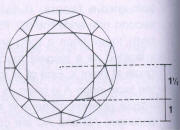69% 1¾:1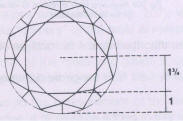72% 2:1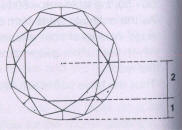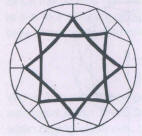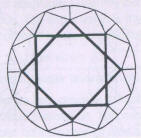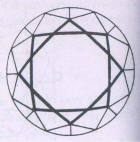Table size = 54 % Table size = 60 % Table size = 66 % Fig 211 The outline of the table alters With the size of the table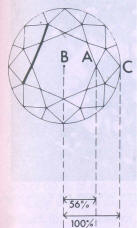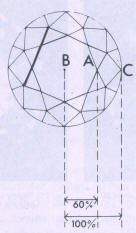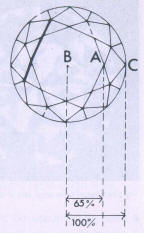Table size = 56 % Table size = 60 % Table size = 65 % Fig 212 Table comparison method: The outline. of the table edge informs about approx. table size
 Table diameter Table comparison method